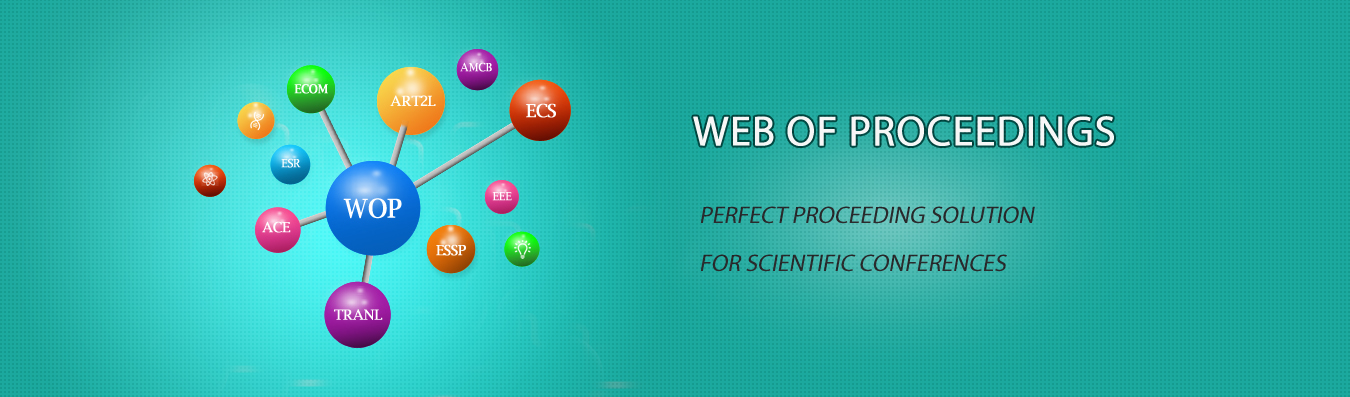The best way to conference proceedings by Francis Academic Press### The Application of Teaching and Modeling in College Mathematics

DOI: 10.25236/icemc.2019.095

Hongfang Wang

Hongfang Wang

#### Abstract

Mathematical modeling is a process of solving practical problems by applying mathematical ideas and methods. This process includes the exploration and discovery of the real world as well as the creation and application of mathematical models. Integrating the thought of mathematical modeling into the teaching content of college mathematics can effectively cultivate the ability of college students to solve practical problems by using mathematical knowledge. Based on the author's learning and practical experience, this work first analyzed the theoretical analysis of mathematical modeling and its important role in college mathematics teaching, and then put forward the application measures of mathematical modeling idea in college mathematics teaching.

#### Keywords

College mathematics, Teaching, Modeling, Teaching reform